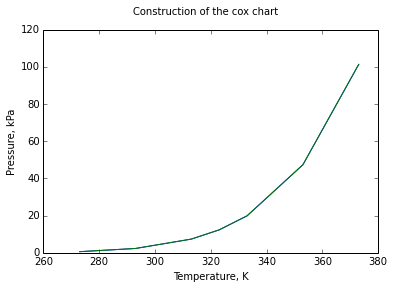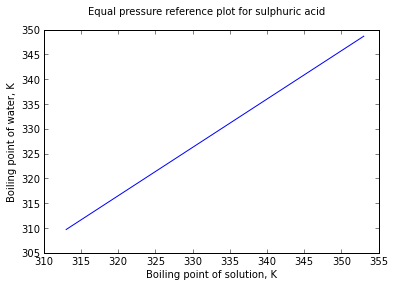# Chapter 6 : Vapour Pressure¶

## Example 6.1 pageno : 133¶

In :
# variables
P = 500.                                #kPa liquid
SV = 0.2813                             #m**3/kg wet steam

# Calculation
Vsaturatedl = 1.093 * 10**-3            #m**3/kg
Vsaturatedv = 0.3747                    #m**3/kg

# let the fraction of vapour be y
#(1-y)*Vsaturatedl + y*Vsaturatedv = SV
#then we get, (1-y)*(1.093*10**-3) + y*(0.3747) = 0.2813
y = (SV - Vsaturatedl)/(Vsaturatedv - Vsaturatedl)
P1 = y * 100
P2 = 100 - P1

# Result
print "Percentage of Vapour = %.f %%"%P1
print "Percentage of Liquid = %.f %%"%P2

Percentage of Vapour = 75 %
Percentage of Liquid = 25 %


## Example 6.2 pageno : 134¶

In :
import math

# Variables
T1 = 363.                           #K water
T2 = 373.                           #K water
P2s = 101.3                         #kPa pressure

# Calculation
J = 2275. * 18                      #kJ/kmol
R = 8.314                           #kJ/kmolK
#ln (P2s/P1s) = J * (1/T1 - 1/T2) / R
P1s = P2s/math.exp(J * (1/T1 - 1/T2) / R)

# Result
print "Vapour pressure of water at 363 K = %.2f kPa"%P1s

Vapour pressure of water at 363 K = 70.41 kPa


## Example 6.3 pageno : 135¶

In :
import math

# Variables
P1s = 194.9                             #kPa acetone
P2s = 8.52                              #kPa pressure
T1 = 353.                               #K acetone
T2 = 273.                               #K acetone
T3 = 300.                               #K dry air
Pair = 101.3                            #kPa dry air
#math.log (P2s/P1s) = J * (1/T1 - 1/T2) / R
#let J / R = L

# Calculation
L = math.log (P2s/P1s)/(1./T1 - 1/T2)
P3s = P1s * math.exp(L * (1./T1 - 1/T3))
Ptotal = P3s + Pair                     #at saturation vapour pressure = partial pressure

# Result
print "(a)Final pressure of the mixture = %.2f kPa"%Ptotal
MP = P3s * 100 / Ptotal
# mole percent = moles of acetone * 100 / total moles
#= Partial pressure of acetone * 100 / total Pressure
print "(b)Mole percent of acetone in the final mixture = %.1f %%"%MP

(a)Final pressure of the mixture = 130.83 kPa
(b)Mole percent of acetone in the final mixture = 22.6 %


## Example 6.4 pageno : 137¶

In :
import math

# Variables
A = 13.8587              # antone constants for n-heptane
B = 2911.32
C = 56.56
T1 = 325.                   #K
#Pressure at normal condition = 101.3kPa
P2 = 101.3                  #kPa

# Calculation
#Antoine equation - lnP = A - B / (T - C)
lnP = A - (B / (T1 - C))
P1 = math.exp(lnP)

# Result
print "(a)Vapour pressure of n-heptane at 325K =  %.2f kPa"%P1
T2 = B/(A - math.log(P2)) + C
print "(b)Normal boiling point of n-heptane = %.2f K"%T2

(a)Vapour pressure of n-heptane at 325K =  20.36 kPa
(b)Normal boiling point of n-heptane = 371.62 K


## Example 6.5 pageno : 140¶

In :
%matplotlib inline

from matplotlib.pyplot import *

# variables
T = [273, 293, 313, 323, 333, 353, 373];
Ps = [0.61, 2.33, 7.37, 12.34, 19.90, 47.35, 101.3];

# Calculation and Result
plot(T,Ps);
T1 = [273, 353]
Ps1 = [8.52, 194.9]
plot(T,Ps);
suptitle("Construction of the cox chart")
xlabel("Temperature, K")
ylabel("Pressure, kPa")
show()

Populating the interactive namespace from numpy and matplotlib

WARNING: pylab import has clobbered these variables: ['draw_if_interactive']
%pylab --no-import-all prevents importing * from pylab and numpy## Example 6.6 page no : 143¶

In :
%matplotlib inline

import math
from matplotlib.pyplot import *

# Variables
Pswater1 = 6.08;            #kPa
T1 = 313.;                  #K

# Calculation
#lnPs = 16.26205 - 3799.887/(T - 46.854)
Tb1 =3799.887/(16.26205 - math.log(Pswater1)) + 46.854;
print "boiling point of water at 6.08kPa vapour pressure = %.2f K"%Tb1
Pswater2 = 39.33;           #kPa
T2 = 353.;                  #K
Tb2 =3799.887/(16.26205 - math.log(Pswater2)) + 46.854;

# Result
print "boiling point of water at 39.33 kPa vapour pressure = %.2f K"%Tb2
Tb = [Tb1 ,Tb2];
T = [T1 ,T2];
plot(T,Tb);
suptitle("Equal pressure reference plot for sulphuric acid")
xlabel("Boiling point of solution, K")
ylabel("Boiling point of water, K")
show()
T3 = 333.;                  #K

#corresponding to T3 on x axis, on y we get
Tb3 = 329.;                 #K
Pswater3 = math.exp(16.26205 - 3799.887/(Tb3 - 46.854));
print "Vapour pressure of solution at 333K %.2f kPa"%Pswater3

# deviation
deviation = .04/16.53 * 100
print "%% deviation = %.2f %%"%deviation

Populating the interactive namespace from numpy and matplotlib
boiling point of water at 6.08kPa vapour pressure = 309.69 K
boiling point of water at 39.33 kPa vapour pressure = 348.67 K

WARNING: pylab import has clobbered these variables: ['draw_if_interactive']
%pylab --no-import-all prevents importing * from pylab and numpyVapour pressure of solution at 333K 16.35 kPa
% deviation = 0.24 %